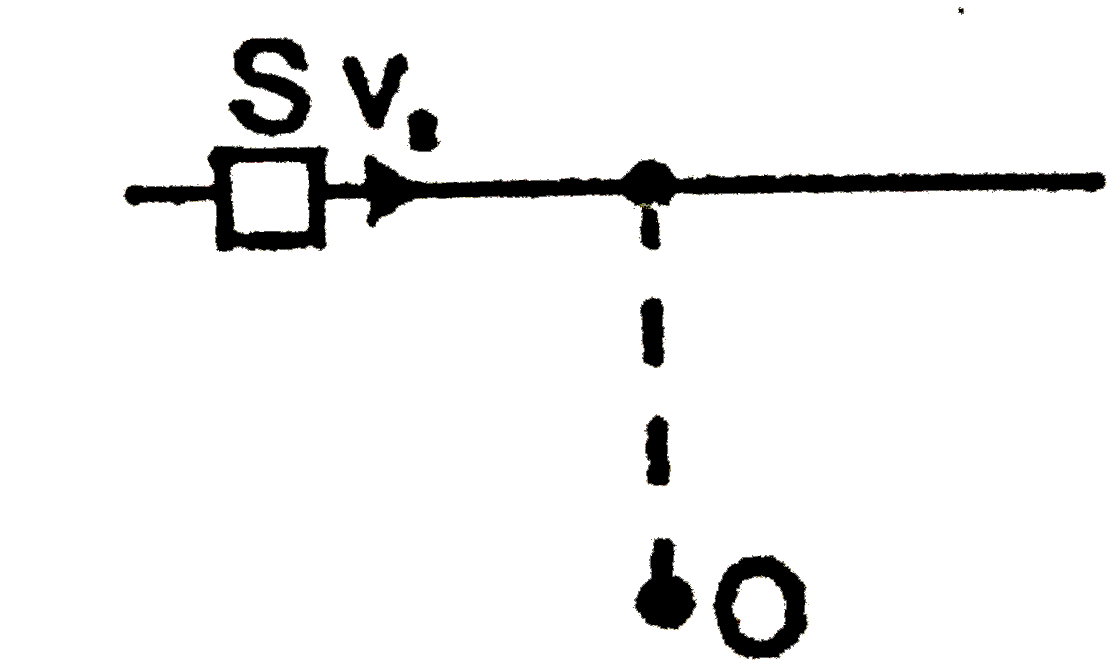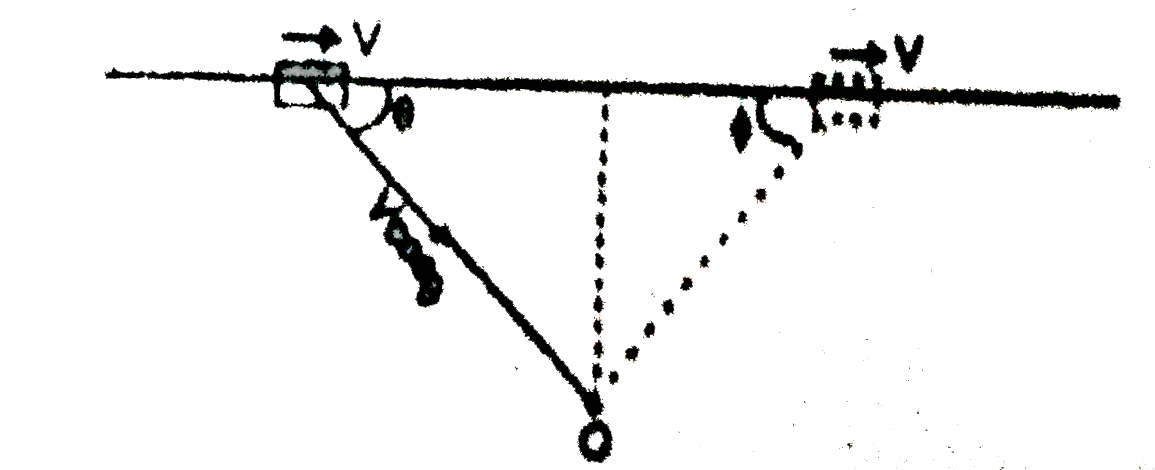# The source (S) of sound is moving constant velocity v_(0) as shown in diagram. An observer O listens to the sound emmited by the source. The observe

40 views
in Physics
closed
The source (S) of sound is moving constant velocity v_(0) as shown in diagram. An observer O listens to the sound emmited by the source. The observed frequency of the soundA. continuously decreases
B. continuously increases
C. first decreases then increases
D. first increases then decreases.

by (85.3k points)
selected

From figure, the velocity of approach (V cos theta) decreases as the source comes closer (as theta increases). And the velocity of separation also increases as phi will decreases. Hence the frequency of should as heard by the observed decreases continuously.